# 在C语言中解释快速排序技术

C语言提供了五种排序技术，如下：

• 冒泡排序（或交换排序）
• 选择排序
• 插入排序（或线性排序）
• 快速排序（或交换排序）
• 归并排序（或外部排序）

## 快速排序

• 步骤1：从数组中选择一个元素，称为枢轴元素。
• 步骤2：将未排序的数组元素分为两个数组。
• 步骤3：如果值小于枢轴元素，则放入第一个子数组；如果值大于枢轴元素，则放入第二个子数组。

• P是枢轴元素。
• L是左指针。
• R是右指针。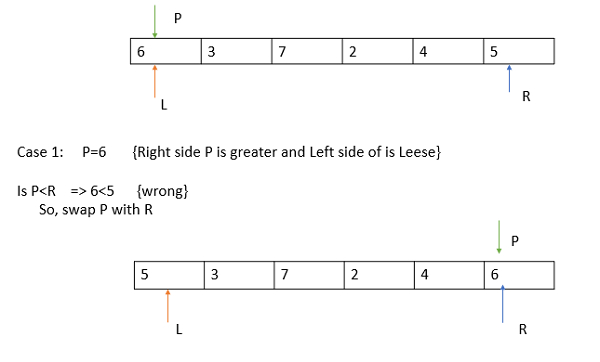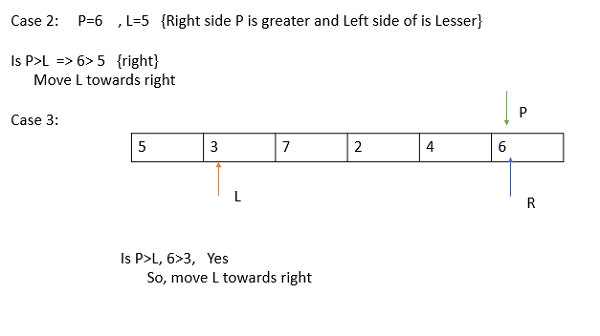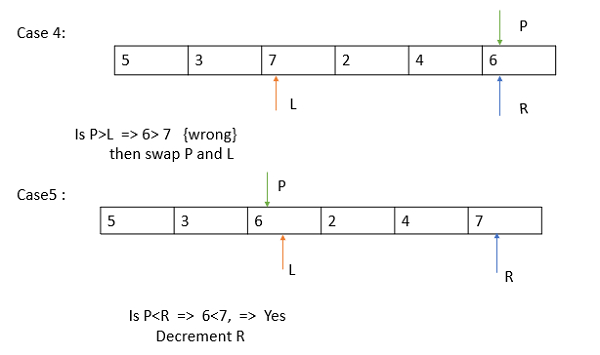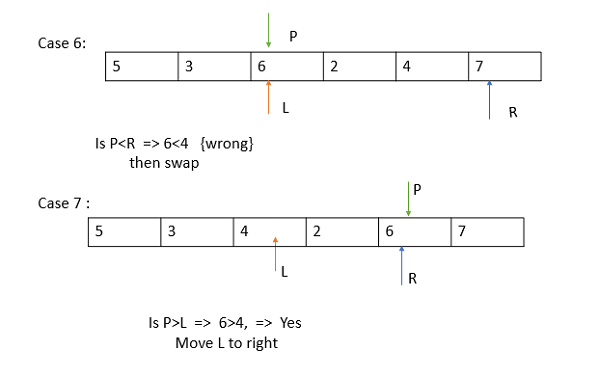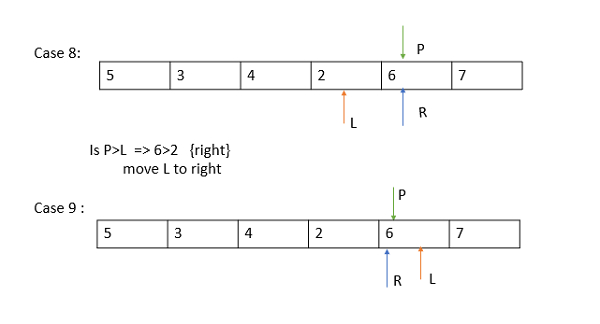• 枢轴元素保持在固定位置。
• 所有左边的元素都较小。
• 右边的元素都大于枢轴。
• 现在将数组分成两个子数组，左边应用快速排序。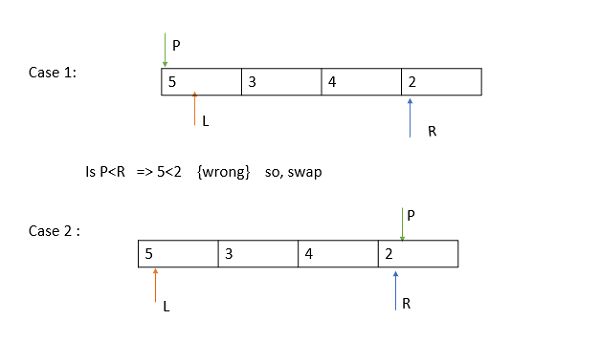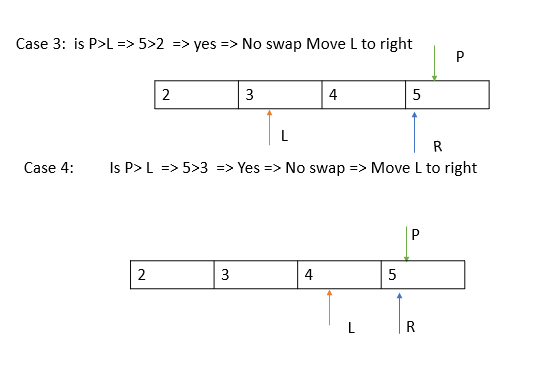• 枢轴元素保持在固定位置。
• 所有左边的元素都较小且已排序。
• 右边的元素较大且已排序。
• 最终排列的列表是2，3，4，5，6，7。

## 示例

#include<stdio.h>
void quicksort(int number,int first,int last){
int i, j, pivot, temp;
if(first<last){
pivot=first;
i=first;
j=last;
while(i<j){
while(number[i]<=number[pivot]&&i<last)
i++;
while(number[j]>number[pivot])
j--;
if(i<j){
temp=number[i];
number[i]=number[j];
number[j]=temp;
}
}
temp=number[pivot];
number[pivot]=number[j];
number[j]=temp;
quicksort(number,first,j-1);
quicksort(number,j+1,last);
}
}
int main(){
int i, count, number;
printf("How many elements are u going to enter?: ");
scanf("%d",&count);
printf("Enter %d elements: ", count);
for(i=0;i<count;i++)
scanf("%d",&number[i]);
quicksort(number,0,count-1);
printf("Order of Sorted elements: ");
for(i=0;i<count;i++)
printf(" %d",number[i]);
return 0;
}


## 输出

How many elements are u going to enter?: 10
Enter 10 elements: 2 3 5 7 1 9 3 8 0 4
Order of Sorted elements: 0 1 2 3 3 4 5 7 8 9


• 微信订阅
• 回顶